Question

describe how light reflecting from a mirror can produce an image. In particular, explain how mirrors can produce images that are larger or smaller than life size, as well as upright or inverted.

1.A larger image is produced when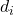>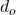A smaller image is produced when<An upright image  is produced when m is positive

An upright image  is produced when m is negative

Explanation:

The mirror equation is given as follows;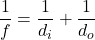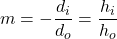For concave mirrors, f = focal length= Image distance from the mirror (-ve= Image is behind the mirror +ve= Image is in front of the mirror)= Object distance from the mirror (-ve= Object is behind the mirror +ve= Object is in front of the mirror)

m = Magnification (-ve m = Inverted image +ve m = upright image)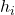= Image height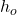= Object height

f = Focal length of the mirror

To produce a larger image>To produce a smaller image<To produce an upright image, m should be positive hence,will be negative or the image will appear behind the mirror

To produce an inverted image, m should be negative hence,will be positive or the image will form in front of the mirror.